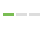# 如何利用TuShare和Python计算移动平均线

``````import pandas as pd
import tushare as ts

stock_data=ts.get_hist_data('300243')
stock_data.sort(inplace=True)
stock_data['60D']=pd.rolling_mean(stock_data['close'],window=60)
stock_data[['close','60D']].plot(figsize=(8,4))``````